Summary
• Wave reflection and transmission
• Optical interference
• Young's interference experiment
Today's joke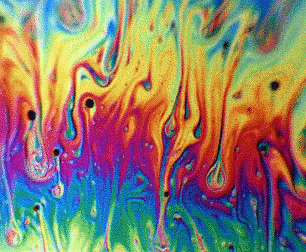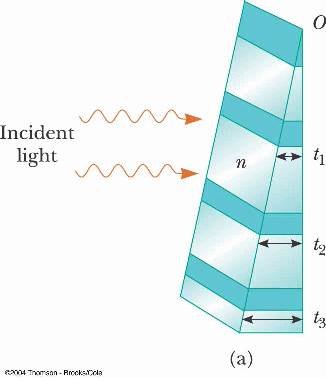• Thin film interference

•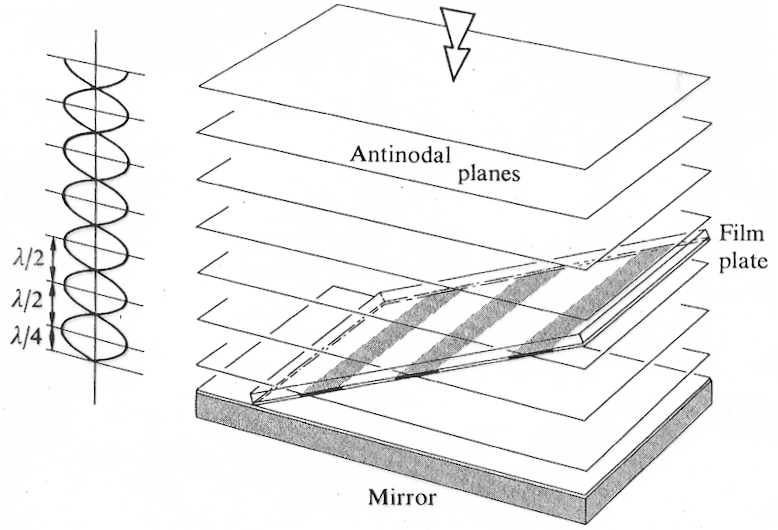• Practice:
• Example #4

Example #5

Example #6

• Prepare:
Read textbook sections 36-1 through 36-3 before the next lecture

PSE6 37.32
An n = 1.25 oil film on wet pavement appears red (λ = 640 nm) and has no blue-green (λ = 512 nm) color. How thick is it?
A. 512 nm
B. 320 nm
C. 256 nm
D. 410 nm

gc6 24.76
How thick should an antireflection coating (n = 1.38) on glass (n = 1.52) be in order to suppress reflection of λ = 515 nm light?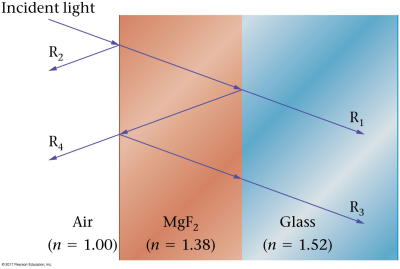A. 93.3 nm
B. 129 nm
C. 187 nm
D. 258 nm

Walker5e 28.36
Monochromatic light with λ = 648 nm shines down on a plano-convex lens lying on a piece of plate glass, as shown below. When viewing from above, one sees a set of concentric dark and bright fringes, referred to as Newton's rings. If the radius of the twelfth dark ring from the center is measured to be 1.56 cm, what is the radius of curvature, R, of the lens?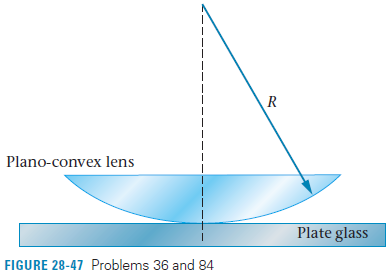A. 1.28 m
B. 31.3 m
C. 74.4 mm
D. 3.89 µm

POP5 27.21
Design a radar antireflection coating for λ = 3.00 cm using a n = 1.50 polymer coating. The thickness should be:
A. 0.25 cm
B. 0.50 cm
C. 1.00 cm
D. 1.50 cm

Walker5e CnEx 28-5
Interference fringes are formed when light shines on an air wedge. Is the point where the glass plates touch a dark fringe or a bright fringe?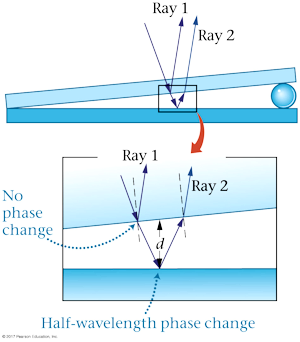A. dark fringe
B. bright fringe
C. It depends on the index of refraction of the glass.

Walker5e RWP Fig 28-21
What is the minimum height h of a "bump" on a DVD that will produce destructive interference when illuminated by half of the λ = 780 nm laser beam (center figure)?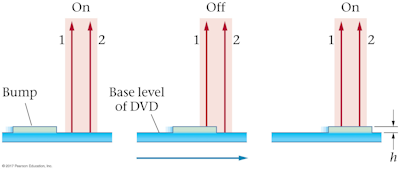A. 1560 nm
B. 780 nm
C. 390 nm
D. 195 nm

A. 512 nm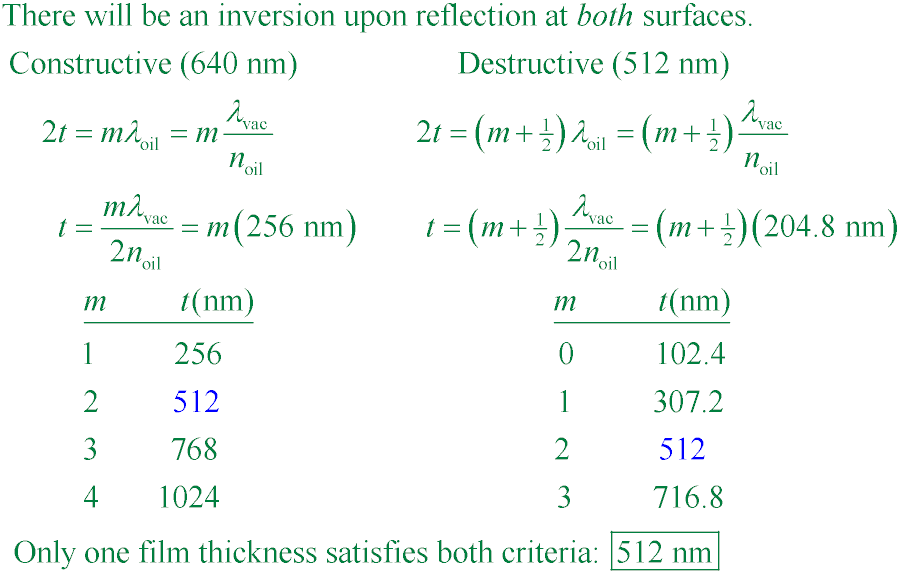A. 93.3 nm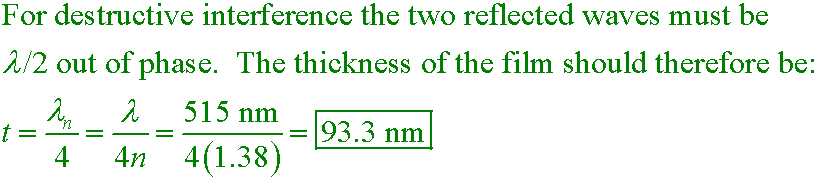B. 31.3 m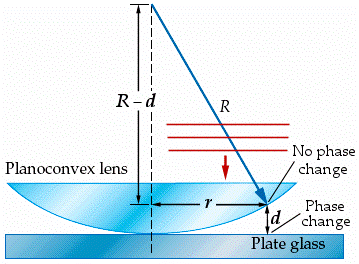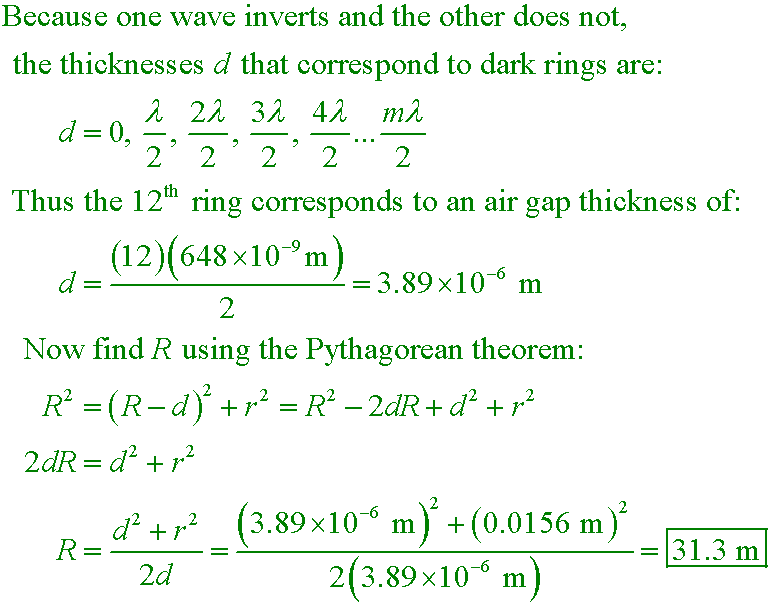Well, it all depends. If you treat the layer as a free-standing film, there will be an inversion on the top layer but not the bottom, so destructive interference will occur when the film is half a wavelength (in the film) thick. The wavelength in the film is λ/n = 2.00 cm, so
C. 1.00 cm
is the best answer. However, if the coating is on top of metal, there will be an inversion at the bottom of the polymer coating, and destructive interference will occur when the film is a quarter wavelength thick, or
B. 0.50 cm
In any case, this anti-reflective coating is easily defeated by choosing a different wavelength. For instance, in the case of a coating on metal, a radar (air) wavelength of 1.50 cm will be brightly reflected because the 0.50-cm-thick film is now half a wavelength thick (the wavelength in the film would be 1.00 cm).A. dark fringe
Ray 1 undergoes no phase change on reflection, whereas ray 2 experiences a 180° phase change due to reflection. It follows, then, that when the path difference 2d approaches zero at the point where the glass plates touch, rays 1 and 2 will cancel with destructive interference.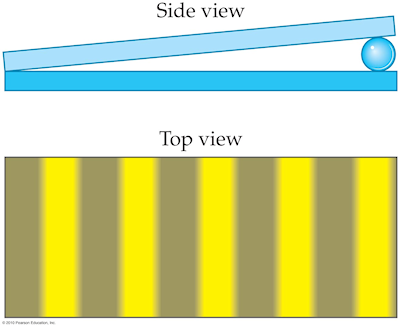D. 195 nm
A bump of minimum height h = λ/4 will force beam 2 to travel half a wavelength farther than beam 1 in the center figure, producing destructive interference between the reflected waves from each beam. The minimum height is thus (780 nm)/4 = 195 nm.-->